# Propositional Logic Methods of Proof Part II Propositional

• Slides: 72Propositional Logic: Methods of Proof (Part II)Propositional Logic: Methods of Proof (Part II) First Lecture Today (Thu 23 Jun) Read Chapter 7. 5 (optional: 7. 6 -7. 8) Second Lecture Today (Thu 23 Jun) Read Chapter 8. 1 -8. 5 Next Lecture (Tue 28 Jun) Review Chapters 8. 3 -8. 5 Read Chapters 9. 1 -9. 2 (optional 9. 5), 13, & 14. 1 -14. 5 (Please read lecture topic material before and after each lecture on that topic)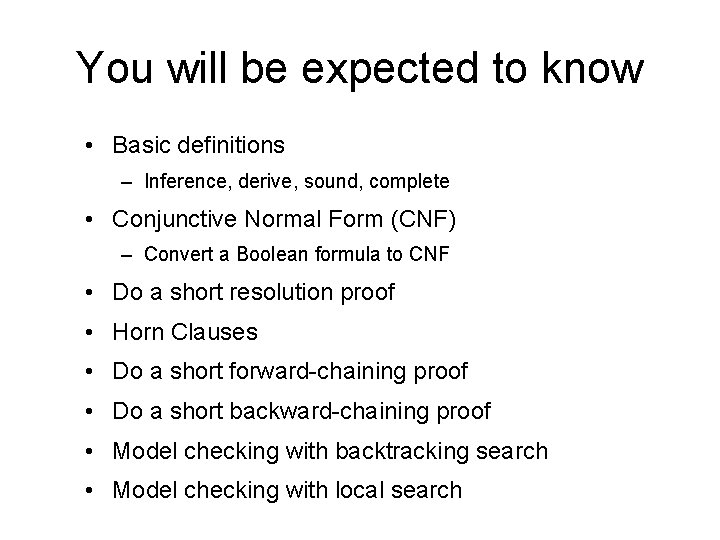You will be expected to know • Basic definitions – Inference, derive, sound, complete • Conjunctive Normal Form (CNF) – Convert a Boolean formula to CNF • Do a short resolution proof • Horn Clauses • Do a short forward-chaining proof • Do a short backward-chaining proof • Model checking with backtracking search • Model checking with local search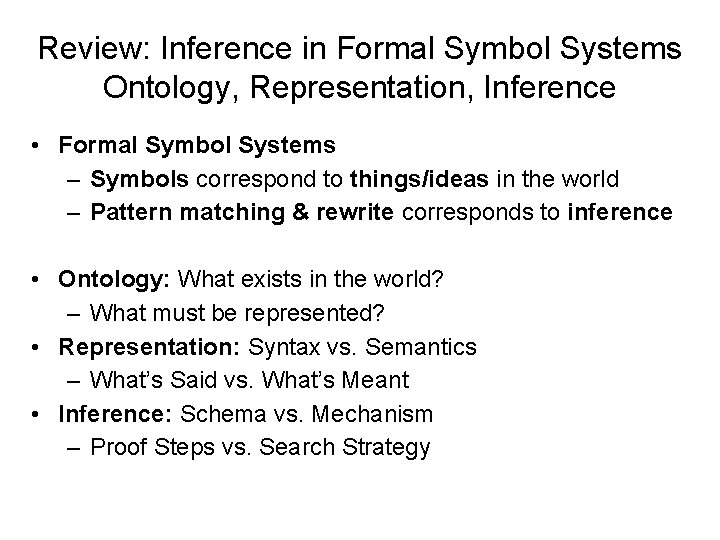Review: Inference in Formal Symbol Systems Ontology, Representation, Inference • Formal Symbol Systems – Symbols correspond to things/ideas in the world – Pattern matching & rewrite corresponds to inference • Ontology: What exists in the world? – What must be represented? • Representation: Syntax vs. Semantics – What’s Said vs. What’s Meant • Inference: Schema vs. Mechanism – Proof Steps vs. Search StrategyOntology: What kind of things exist in the world? What do we need to describe and reason about? Review Reasoning Representation ---------A Formal Symbol System Syntax ----What is said Semantics ------What it means Preceding lecture Inference ----------Formal Pattern Matching Schema ------Rules of Inference This lecture Execution ------Search StrategyReview • Definitions: – Syntax, Semantics, Sentences, Propositions, Entails, Follows, Derives, Inference, Sound, Complete, Model, Satisfiable, Valid (or Tautology) • Syntactic Transformations: – E. g. , (A ⇒ B) ⇔ (¬A ∨ B) • Semantic Transformations: – E. g. , (KB |= α) ≡ (|= (KB ⇒ α) ) • Truth Tables – Negation, Conjunction, Disjunction, Implication, Equivalence (Biconditional) – Inference by Model EnumerationReview: Schematic perspective If KB is true in the real world, then any sentence α entailed by KB is also true in the real world.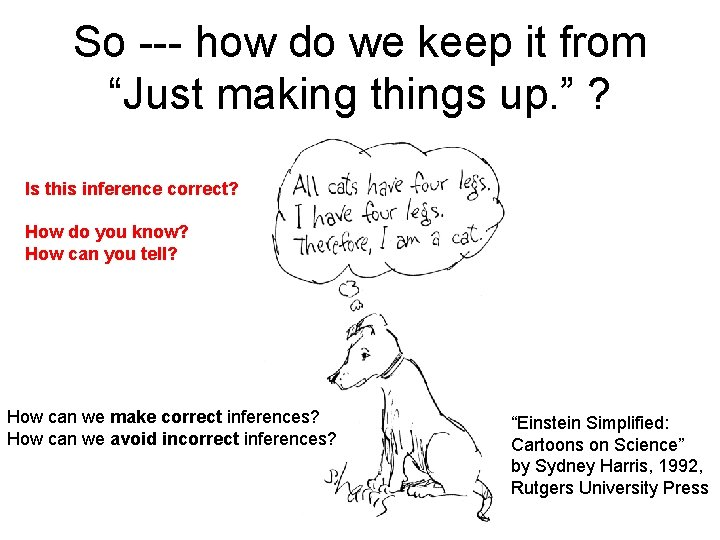So --- how do we keep it from “Just making things up. ” ? Is this inference correct? How do you know? How can you tell? How can we make correct inferences? How can we avoid incorrect inferences? “Einstein Simplified: Cartoons on Science” by Sydney Harris, 1992, Rutgers University Press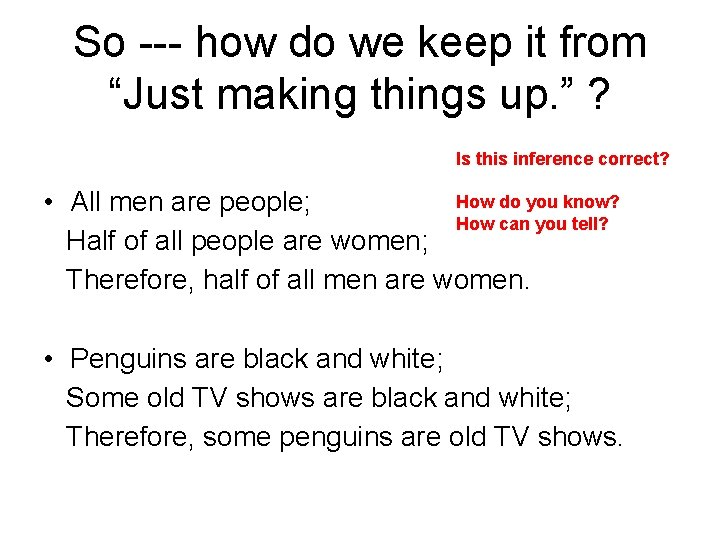So --- how do we keep it from “Just making things up. ” ? Is this inference correct? How do you know? • All men are people; How can you tell? Half of all people are women; Therefore, half of all men are women. • Penguins are black and white; Some old TV shows are black and white; Therefore, some penguins are old TV shows.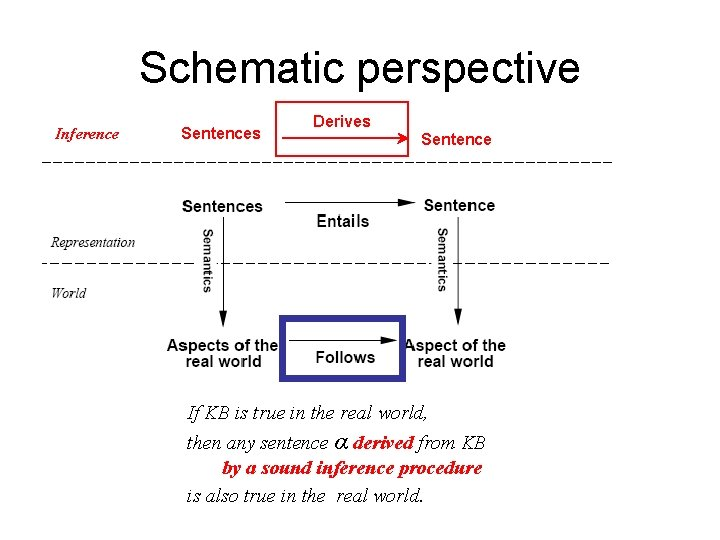Schematic perspective Inference Sentences Derives Sentence If KB is true in the real world, then any sentence α derived from KB by a sound inference procedure is also true in the real world.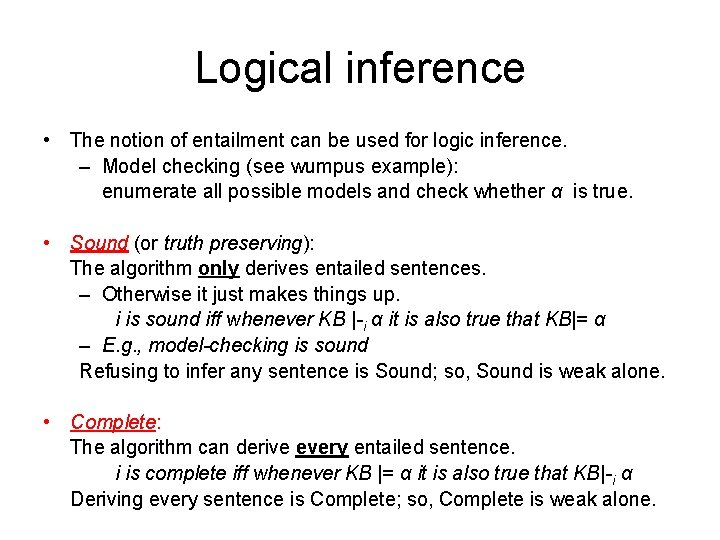Logical inference • The notion of entailment can be used for logic inference. – Model checking (see wumpus example): enumerate all possible models and check whether α is true. • Sound (or truth preserving): The algorithm only derives entailed sentences. – Otherwise it just makes things up. i is sound iff whenever KB |-i α it is also true that KB|= α – E. g. , model-checking is sound Refusing to infer any sentence is Sound; so, Sound is weak alone. • Complete: The algorithm can derive every entailed sentence. i is complete iff whenever KB |= α it is also true that KB|-i α Deriving every sentence is Complete; so, Complete is weak alone.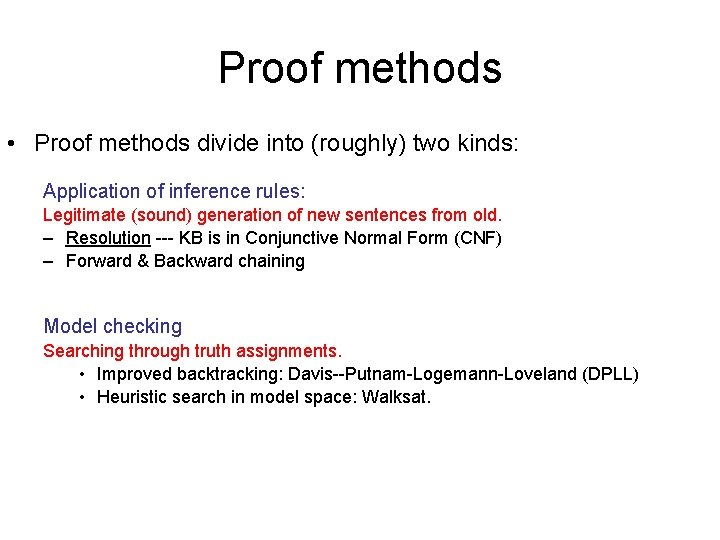Proof methods • Proof methods divide into (roughly) two kinds: Application of inference rules: Legitimate (sound) generation of new sentences from old. – Resolution --- KB is in Conjunctive Normal Form (CNF) – Forward & Backward chaining Model checking Searching through truth assignments. • Improved backtracking: Davis--Putnam-Logemann-Loveland (DPLL) • Heuristic search in model space: Walksat.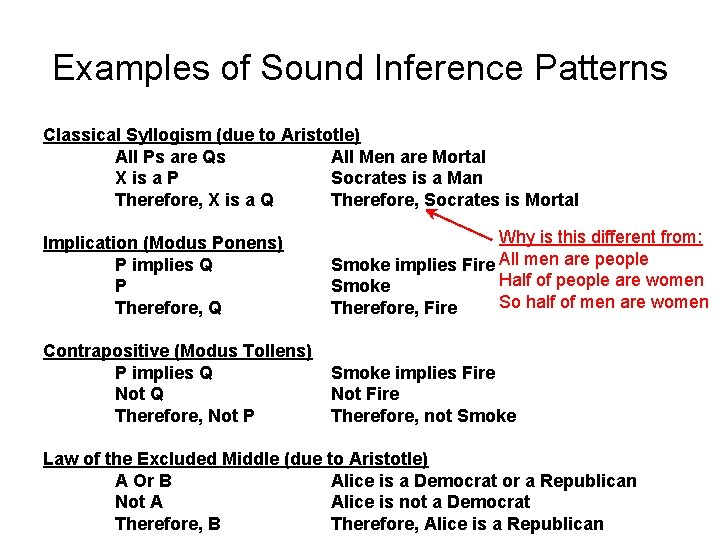Examples of Sound Inference Patterns Classical Syllogism (due to Aristotle) All Ps are Qs All Men are Mortal X is a P Socrates is a Man Therefore, X is a Q Therefore, Socrates is Mortal Implication (Modus Ponens) P implies Q P Therefore, Q Why is this different from: Smoke implies Fire All men are people Half of people are women Smoke So half of men are women Therefore, Fire Contrapositive (Modus Tollens) P implies Q Not Q Therefore, Not P Smoke implies Fire Not Fire Therefore, not Smoke Law of the Excluded Middle (due to Aristotle) A Or B Alice is a Democrat or a Republican Not A Alice is not a Democrat Therefore, B Therefore, Alice is a RepublicanInference by Resolution • KB is represented in CNF – KB = AND of all the sentences in KB – KB sentence = clause = OR of literals – Literal = propositional symbol or its negation • Find two clauses in KB, one of which contains a literal and the other its negation • Cancel the literal and its negation • Bundle everything else into a new clause • Add the new clause to KBConjunctive Normal Form (CNF) • Boolean formulae are central to CS – Boolean logic is the way our discipline works • Two canonical Boolean formulae representations: Clause – CNF = Conjunctive Normal Form • A conjunct of disjuncts = (AND (OR …) ) • “…” = a list of literals (= a variable or its negation) • CNF is used by Resolution Theorem Proving – DNF = Disjunctive Normal Form Term • A disjunct of conjuncts = (OR (AND …) ) • DNF is used by Decision Trees in Machine Learning • Can convert any Boolean formula to CNF or DNF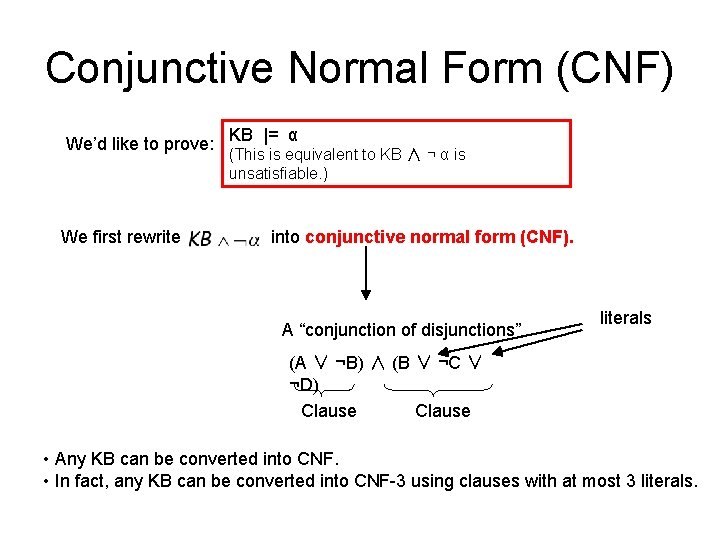Conjunctive Normal Form (CNF) We’d like to prove: We first rewrite KB |= α (This is equivalent to KB ∧ ¬ α is unsatisfiable. ) into conjunctive normal form (CNF). A “conjunction of disjunctions” literals (A ∨ ¬B) ∧ (B ∨ ¬C ∨ ¬D) Clause • Any KB can be converted into CNF. • In fact, any KB can be converted into CNF-3 using clauses with at most 3 literals.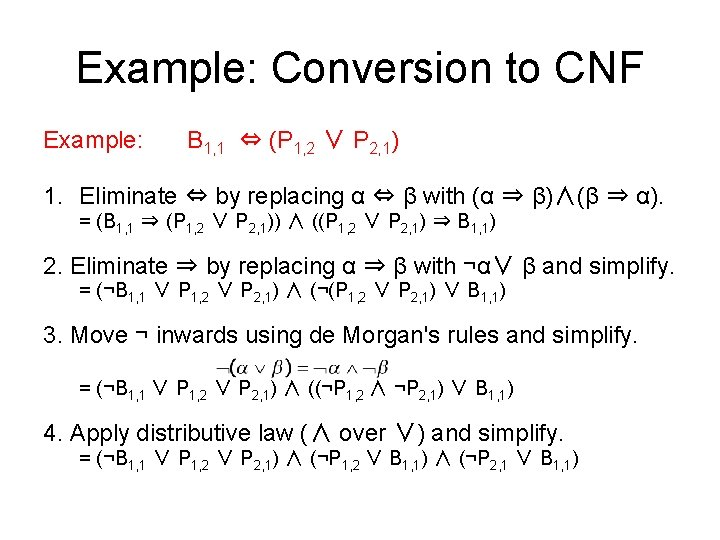Example: Conversion to CNF Example: B 1, 1 ⇔ (P 1, 2 ∨ P 2, 1) 1. Eliminate ⇔ by replacing α ⇔ β with (α ⇒ β)∧(β ⇒ α). = (B 1, 1 ⇒ (P 1, 2 ∨ P 2, 1)) ∧ ((P 1, 2 ∨ P 2, 1) ⇒ B 1, 1) 2. Eliminate ⇒ by replacing α ⇒ β with ¬α∨ β and simplify. = (¬B 1, 1 ∨ P 1, 2 ∨ P 2, 1) ∧ (¬(P 1, 2 ∨ P 2, 1) ∨ B 1, 1) 3. Move ¬ inwards using de Morgan's rules and simplify. = (¬B 1, 1 ∨ P 1, 2 ∨ P 2, 1) ∧ ((¬P 1, 2 ∧ ¬P 2, 1) ∨ B 1, 1) 4. Apply distributive law (∧ over ∨) and simplify. = (¬B 1, 1 ∨ P 1, 2 ∨ P 2, 1) ∧ (¬P 1, 2 ∨ B 1, 1) ∧ (¬P 2, 1 ∨ B 1, 1)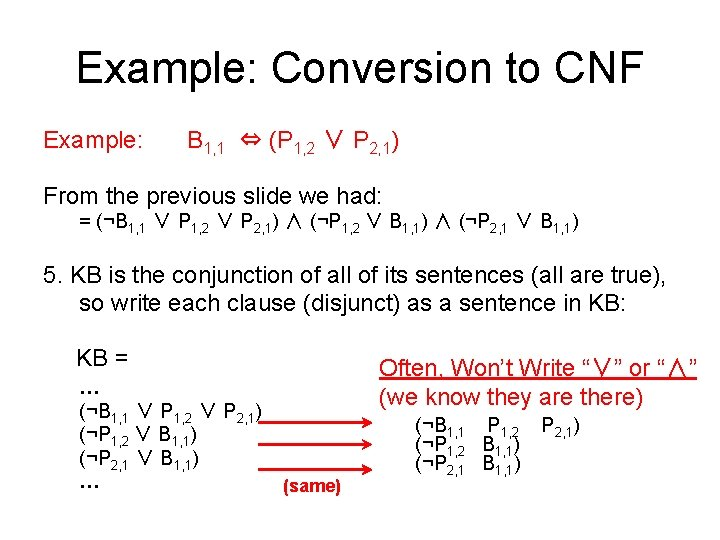Example: Conversion to CNF Example: B 1, 1 ⇔ (P 1, 2 ∨ P 2, 1) From the previous slide we had: = (¬B 1, 1 ∨ P 1, 2 ∨ P 2, 1) ∧ (¬P 1, 2 ∨ B 1, 1) ∧ (¬P 2, 1 ∨ B 1, 1) 5. KB is the conjunction of all of its sentences (all are true), so write each clause (disjunct) as a sentence in KB: KB = … (¬B 1, 1 ∨ P 1, 2 ∨ P 2, 1) (¬P 1, 2 ∨ B 1, 1) (¬P 2, 1 ∨ B 1, 1) … Often, Won’t Write “∨” or “∧” (we know they are there) (same) (¬B 1, 1 P 1, 2 (¬P 1, 2 B 1, 1) (¬P 2, 1 B 1, 1) P 2, 1)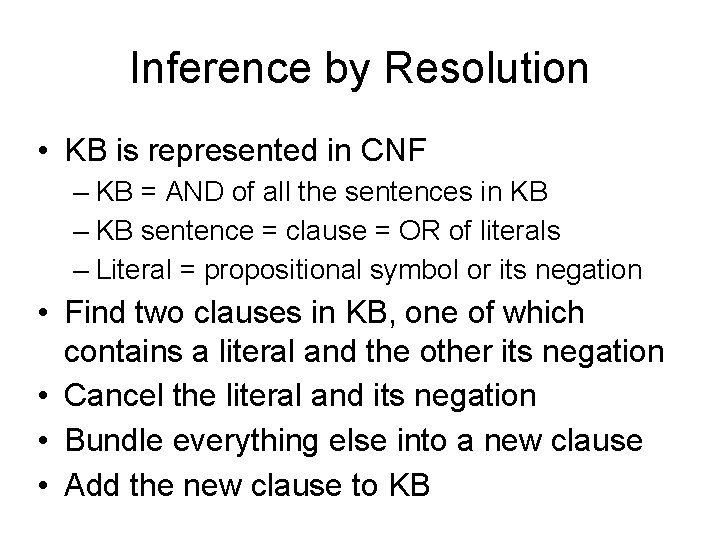Inference by Resolution • KB is represented in CNF – KB = AND of all the sentences in KB – KB sentence = clause = OR of literals – Literal = propositional symbol or its negation • Find two clauses in KB, one of which contains a literal and the other its negation • Cancel the literal and its negation • Bundle everything else into a new clause • Add the new clause to KBResolution = Efficient Implication Recall that (A => B) = ( (NOT A) OR B) and so: (Y OR X) = ( (NOT X) => Y) ( (NOT Y) OR Z) = (Y => Z) which yields: ( (Y OR X) AND ( (NOT Y) OR Z) ) = ( (NOT X) => Z) = (X OR Z) (OR A B C D) ->Same -> (OR ¬A E F G) ->Same -> --------------(OR B C D E F G) (NOT (OR B C D)) => A A => (OR E F G) --------------------------(NOT (OR B C D)) => (OR E F G) --------------------------(OR B C D E F G) Recall: All clauses in KB are conjoined by an implicit AND (= CNF representation).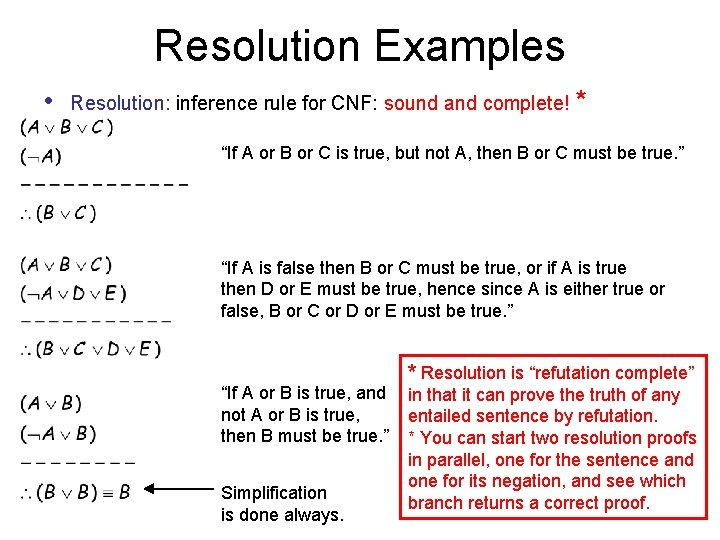Resolution Examples • Resolution: inference rule for CNF: sound and complete! * “If A or B or C is true, but not A, then B or C must be true. ” “If A is false then B or C must be true, or if A is true then D or E must be true, hence since A is either true or false, B or C or D or E must be true. ” “If A or B is true, and not A or B is true, then B must be true. ” Simplification is done always. * Resolution is “refutation complete” in that it can prove the truth of any entailed sentence by refutation. * You can start two resolution proofs in parallel, one for the sentence and one for its negation, and see which branch returns a correct proof.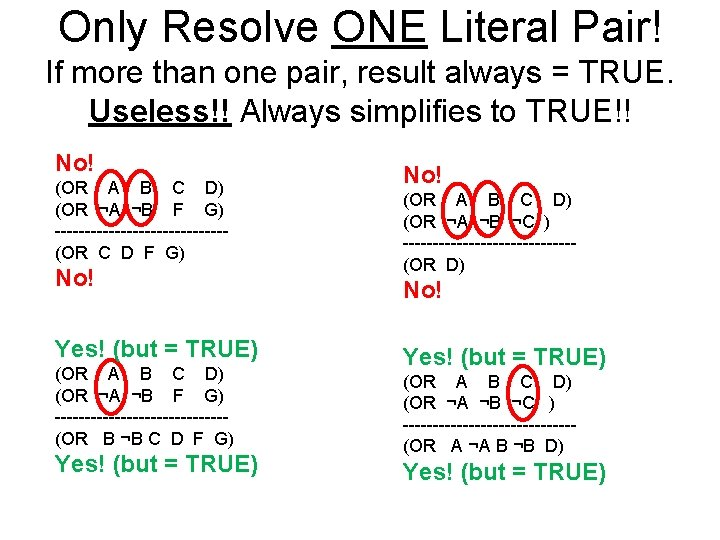Only Resolve ONE Literal Pair! If more than one pair, result always = TRUE. Useless!! Always simplifies to TRUE!! No! (OR A B C D) (OR ¬A ¬B F G) --------------(OR C D F G) No! Yes! (but = TRUE) (OR A B C D) (OR ¬A ¬B F G) --------------(OR B ¬B C D F G) Yes! (but = TRUE) No! (OR A B C D) (OR ¬A ¬B ¬C ) --------------(OR D) No! Yes! (but = TRUE) (OR A B C D) (OR ¬A ¬B ¬C ) --------------(OR A ¬A B ¬B D) Yes! (but = TRUE)Resolution Algorithm • The resolution algorithm tries to prove: • • Generate all new sentences from KB and the (negated) query. One of two things can happen: 1. We find which is unsatisfiable. I. e. we can entail the query. 1. We find no contradiction: there is a model that satisfies the sentence (non-trivial) and hence we cannot entail the query.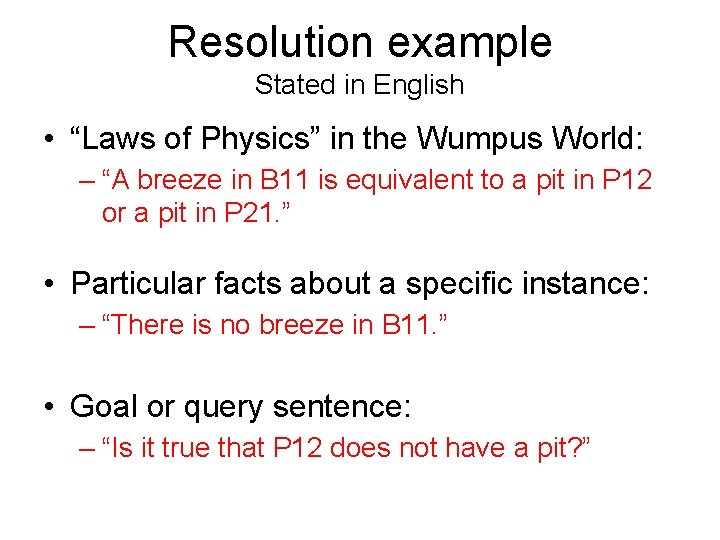Resolution example Stated in English • “Laws of Physics” in the Wumpus World: – “A breeze in B 11 is equivalent to a pit in P 12 or a pit in P 21. ” • Particular facts about a specific instance: – “There is no breeze in B 11. ” • Goal or query sentence: – “Is it true that P 12 does not have a pit? ”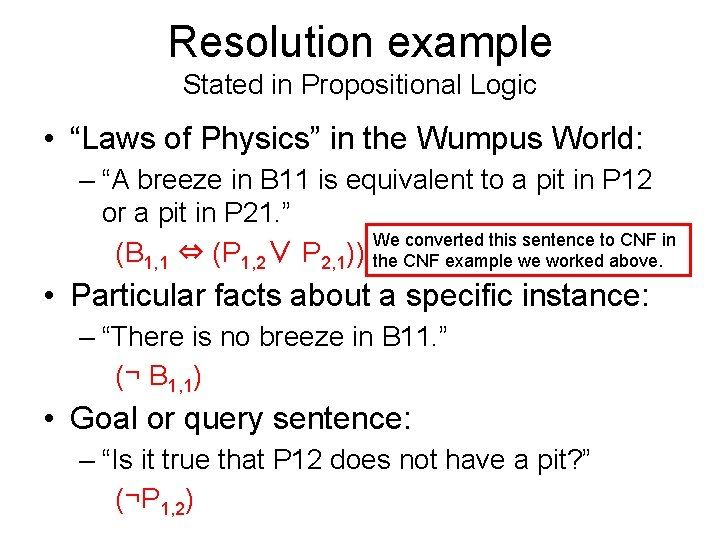Resolution example Stated in Propositional Logic • “Laws of Physics” in the Wumpus World: – “A breeze in B 11 is equivalent to a pit in P 12 or a pit in P 21. ” We converted this sentence to CNF in (B 1, 1 ⇔ (P 1, 2∨ P 2, 1)) the CNF example we worked above. • Particular facts about a specific instance: – “There is no breeze in B 11. ” (¬ B 1, 1) • Goal or query sentence: – “Is it true that P 12 does not have a pit? ” (¬P 1, 2)Resolution example Resulting Knowledge Base stated in CNF • “Laws of Physics” in the Wumpus World: (¬B 1, 1 P 1, 2 (¬P 1, 2 B 1, 1) (¬P 2, 1 B 1, 1) P 2, 1) • Particular facts about a specific instance: (¬ B 1, 1) • Negated goal or query sentence: (P 1, 2)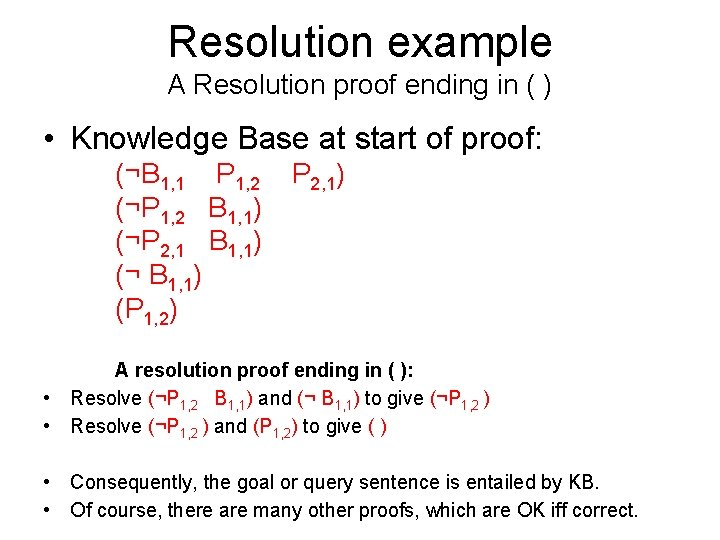Resolution example A Resolution proof ending in ( ) • Knowledge Base at start of proof: (¬B 1, 1 P 1, 2 (¬P 1, 2 B 1, 1) (¬P 2, 1 B 1, 1) (¬ B 1, 1) (P 1, 2) P 2, 1) A resolution proof ending in ( ): • Resolve (¬P 1, 2 B 1, 1) and (¬ B 1, 1) to give (¬P 1, 2 ) • Resolve (¬P 1, 2 ) and (P 1, 2) to give ( ) • Consequently, the goal or query sentence is entailed by KB. • Of course, there are many other proofs, which are OK iff correct.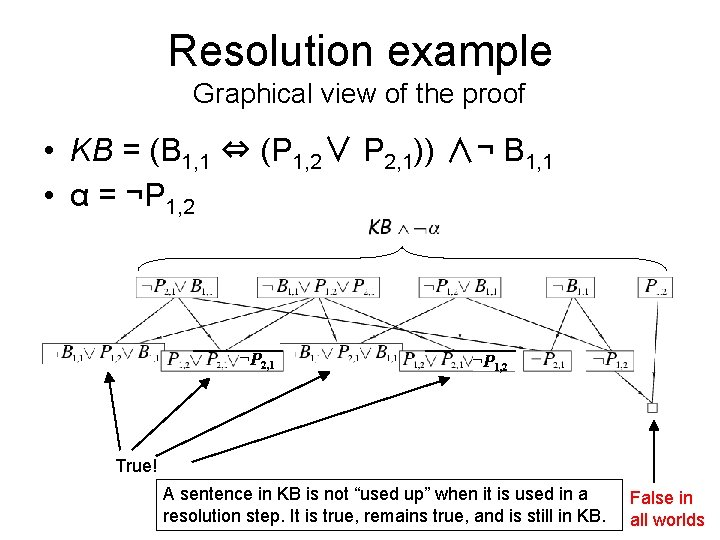Resolution example Graphical view of the proof • KB = (B 1, 1 ⇔ (P 1, 2∨ P 2, 1)) ∧¬ B 1, 1 • α = ¬P 1, 2 ¬P 2, 1 ¬P 1, 2 True! A sentence in KB is not “used up” when it is used in a resolution step. It is true, remains true, and is still in KB. False in all worldsDetailed Resolution Proof Example • In words: If the unicorn is mythical, then it is immortal, but if it is not mythical, then it is a mortal mammal. If the unicorn is either immortal or a mammal, then it is horned. The unicorn is magical if it is horned. Prove that the unicorn is both magical and horned. Problem 7. 2, R&N page 280. (Adapted from Barwise and Etchemendy, 1993. ) Note for non-native-English speakers: immortal = not mortalDetailed Resolution Proof Example • In words: If the unicorn is mythical, then it is immortal, but if it is not mythical, then it is a mortal mammal. If the unicorn is either immortal or a mammal, then it is horned. The unicorn is magical if it is horned. Prove that the unicorn is both magical and horned. • First, Ontology: What do we need to describe and reason about? • Use these propositional variables (“immortal” = “not mortal”): Y = unicorn is m. Ythical R = unicorn is mo. Rtal M = unicorn is a ma. Mmal H = unicorn is Horned G = unicorn is ma. Gical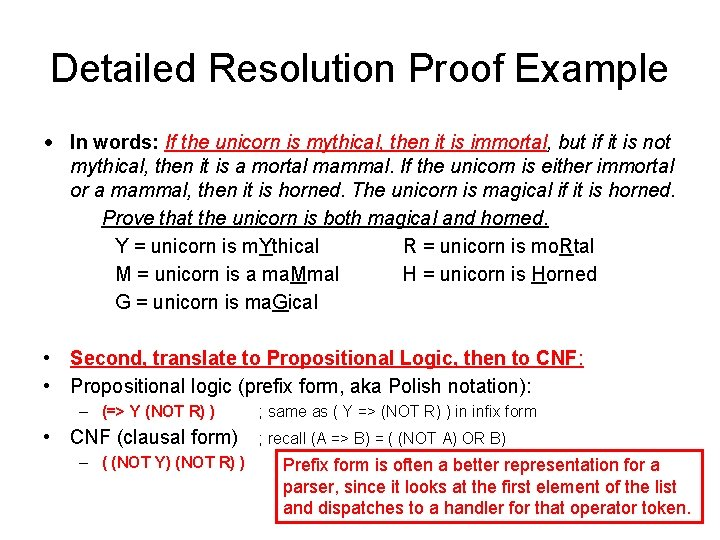Detailed Resolution Proof Example • In words: If the unicorn is mythical, then it is immortal, but if it is not mythical, then it is a mortal mammal. If the unicorn is either immortal or a mammal, then it is horned. The unicorn is magical if it is horned. Prove that the unicorn is both magical and horned. Y = unicorn is m. Ythical R = unicorn is mo. Rtal M = unicorn is a ma. Mmal H = unicorn is Horned G = unicorn is ma. Gical • Second, translate to Propositional Logic, then to CNF: • Propositional logic (prefix form, aka Polish notation): – (=> Y (NOT R) ) • CNF (clausal form) – ( (NOT Y) (NOT R) ) ; same as ( Y => (NOT R) ) in infix form ; recall (A => B) = ( (NOT A) OR B) Prefix form is often a better representation for a parser, since it looks at the first element of the list and dispatches to a handler for that operator token.Detailed Resolution Proof Example • In words: If the unicorn is mythical, then it is immortal, but if it is not mythical, then it is a mortal mammal. If the unicorn is either immortal or a mammal, then it is horned. The unicorn is magical if it is horned. Prove that the unicorn is both magical and horned. Y = unicorn is m. Ythical R = unicorn is mo. Rtal M = unicorn is a ma. Mmal H = unicorn is Horned G = unicorn is ma. Gical • Second, translate to Propositional Logic, then to CNF: • Propositional logic (prefix form): – (=> (NOT Y) (AND R M) ) • CNF (clausal form) – (M Y) – (R Y) ; same as ( (NOT Y) => (R AND M) ) in infix form If you ever have to do this “for real” you will likely invent a new domain language that allows you to state important properties of the domain --- then parse that into propositional logic, and then CNF.Detailed Resolution Proof Example • In words: If the unicorn is mythical, then it is immortal, but if it is not mythical, then it is a mortal mammal. If the unicorn is either immortal or a mammal, then it is horned. The unicorn is magical if it is horned. Prove that the unicorn is both magical and horned. Y = unicorn is m. Ythical R = unicorn is mo. Rtal M = unicorn is a ma. Mmal H = unicorn is Horned G = unicorn is ma. Gical • Second, translate to Propositional Logic, then to CNF: • Propositional logic (prefix form): – (=> (OR (NOT R) M) H) • CNF (clausal form) – (H (NOT M) ) – (H R) ; same as ( (Not R) OR M) => H in infix form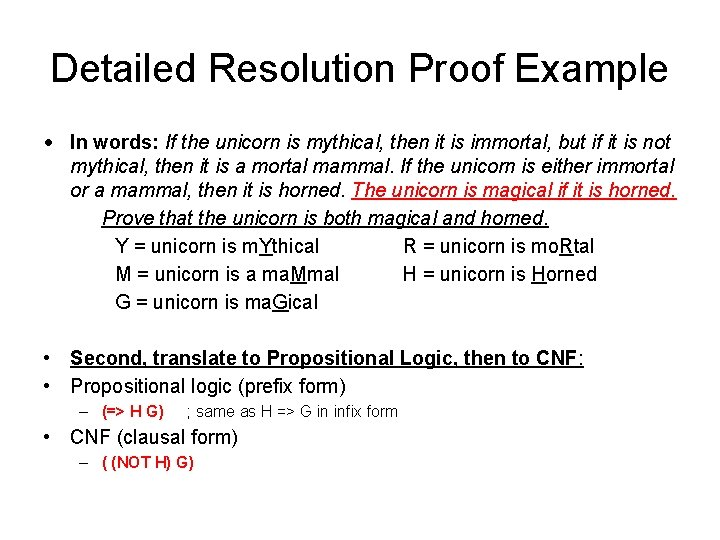Detailed Resolution Proof Example • In words: If the unicorn is mythical, then it is immortal, but if it is not mythical, then it is a mortal mammal. If the unicorn is either immortal or a mammal, then it is horned. The unicorn is magical if it is horned. Prove that the unicorn is both magical and horned. Y = unicorn is m. Ythical R = unicorn is mo. Rtal M = unicorn is a ma. Mmal H = unicorn is Horned G = unicorn is ma. Gical • Second, translate to Propositional Logic, then to CNF: • Propositional logic (prefix form) – (=> H G) ; same as H => G in infix form • CNF (clausal form) – ( (NOT H) G)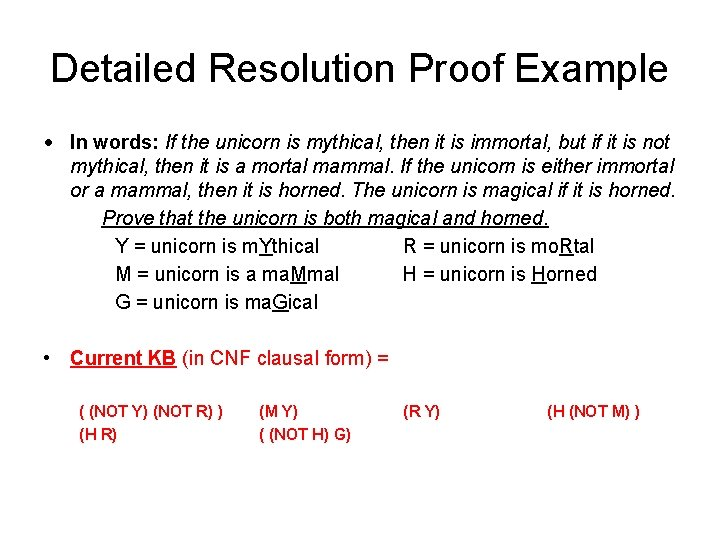Detailed Resolution Proof Example • In words: If the unicorn is mythical, then it is immortal, but if it is not mythical, then it is a mortal mammal. If the unicorn is either immortal or a mammal, then it is horned. The unicorn is magical if it is horned. Prove that the unicorn is both magical and horned. Y = unicorn is m. Ythical R = unicorn is mo. Rtal M = unicorn is a ma. Mmal H = unicorn is Horned G = unicorn is ma. Gical • Current KB (in CNF clausal form) = ( (NOT Y) (NOT R) ) (H R) (M Y) ( (NOT H) G) (R Y) (H (NOT M) )Detailed Resolution Proof Example • In words: If the unicorn is mythical, then it is immortal, but if it is not mythical, then it is a mortal mammal. If the unicorn is either immortal or a mammal, then it is horned. The unicorn is magical if it is horned. Prove that the unicorn is both magical and horned. Y = unicorn is m. Ythical R = unicorn is mo. Rtal M = unicorn is a ma. Mmal H = unicorn is Horned G = unicorn is ma. Gical • Third, negated goal to Propositional Logic, then to CNF: • Goal sentence in propositional logic (prefix form) – (AND H G) ; same as H AND G in infix form • Negated goal sentence in propositional logic (prefix form) – (NOT (AND H G) ) = (OR (NOT H) (NOT G) ) • CNF (clausal form) – ( (NOT G) (NOT H) )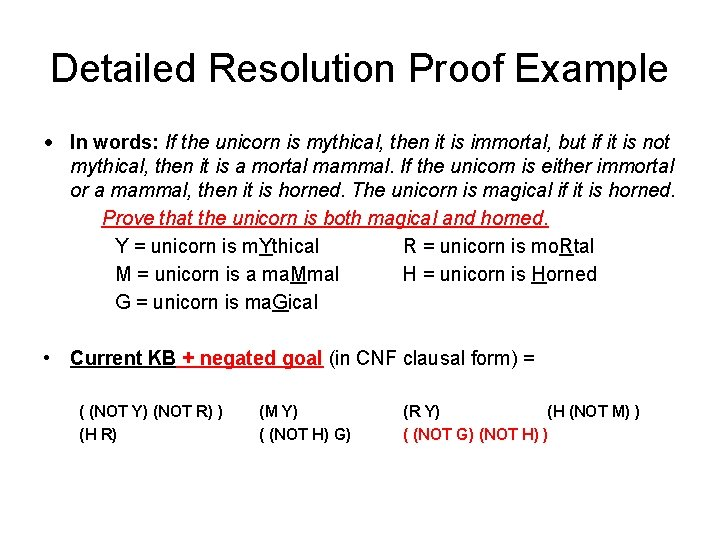Detailed Resolution Proof Example • In words: If the unicorn is mythical, then it is immortal, but if it is not mythical, then it is a mortal mammal. If the unicorn is either immortal or a mammal, then it is horned. The unicorn is magical if it is horned. Prove that the unicorn is both magical and horned. Y = unicorn is m. Ythical R = unicorn is mo. Rtal M = unicorn is a ma. Mmal H = unicorn is Horned G = unicorn is ma. Gical • Current KB + negated goal (in CNF clausal form) = ( (NOT Y) (NOT R) ) (H R) (M Y) ( (NOT H) G) (R Y) (H (NOT M) ) ( (NOT G) (NOT H) )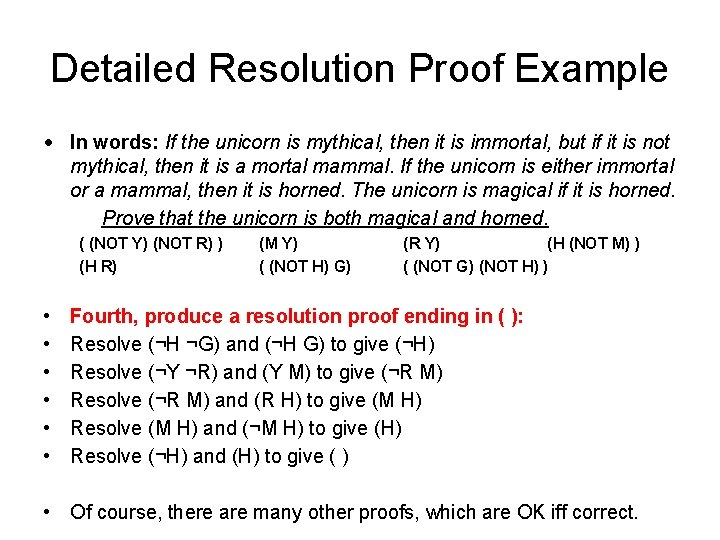Detailed Resolution Proof Example • In words: If the unicorn is mythical, then it is immortal, but if it is not mythical, then it is a mortal mammal. If the unicorn is either immortal or a mammal, then it is horned. The unicorn is magical if it is horned. Prove that the unicorn is both magical and horned. ( (NOT Y) (NOT R) ) (H R) • • • (M Y) ( (NOT H) G) (R Y) (H (NOT M) ) ( (NOT G) (NOT H) ) Fourth, produce a resolution proof ending in ( ): Resolve (¬H ¬G) and (¬H G) to give (¬H) Resolve (¬Y ¬R) and (Y M) to give (¬R M) Resolve (¬R M) and (R H) to give (M H) Resolve (M H) and (¬M H) to give (H) Resolve (¬H) and (H) to give ( ) • Of course, there are many other proofs, which are OK iff correct.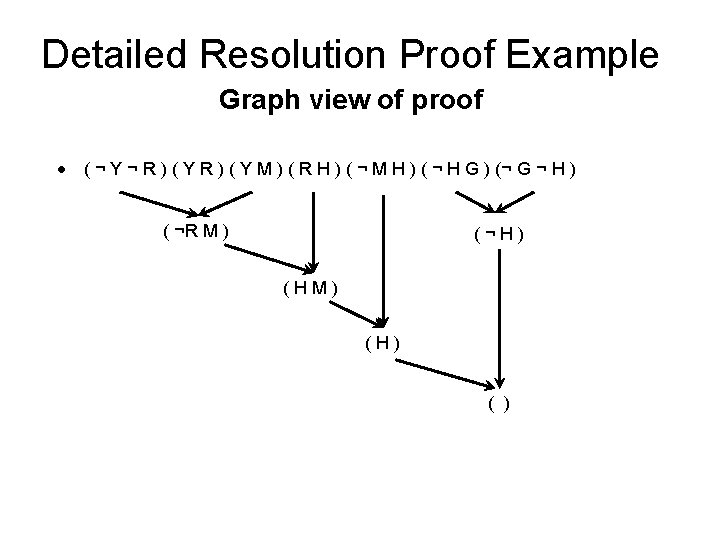Detailed Resolution Proof Example Graph view of proof • ( ¬ Y ¬ R ) ( Y M ) ( R H ) ( ¬ M H ) ( ¬ H G ) (¬ G ¬ H ) ( ¬R M ) (¬H) (HM) (H) ( )Detailed Resolution Proof Example Graph view of a different proof • ( ¬ Y ¬ R ) ( Y M ) ( R H ) ( ¬ M H ) ( ¬ H G ) (¬ G ¬ H ) (¬H) (¬M) (Y) (¬R) (H) ( )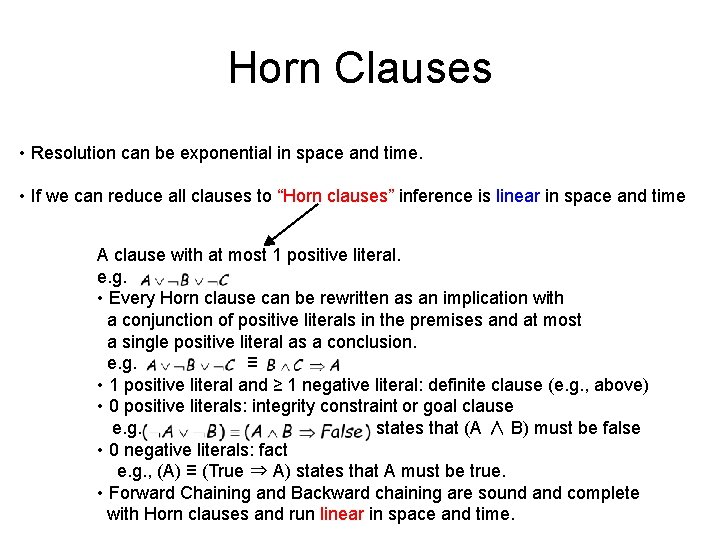Horn Clauses • Resolution can be exponential in space and time. • If we can reduce all clauses to “Horn clauses” inference is linear in space and time A clause with at most 1 positive literal. e. g. • Every Horn clause can be rewritten as an implication with a conjunction of positive literals in the premises and at most a single positive literal as a conclusion. e. g. ≡ • 1 positive literal and ≥ 1 negative literal: definite clause (e. g. , above) • 0 positive literals: integrity constraint or goal clause e. g. states that (A ∧ B) must be false • 0 negative literals: fact e. g. , (A) ≡ (True ⇒ A) states that A must be true. • Forward Chaining and Backward chaining are sound and complete with Horn clauses and run linear in space and time.Forward chaining (FC) • Idea: fire any rule whose premises are satisfied in the KB, add its conclusion to the KB, until query is found. • This proves that is true in all possible worlds (i. e. trivial), and hence it proves entailment. AND gate OR gate • Forward chaining is sound and complete for Horn KBForward chaining example “OR” Gate “AND” gateForward chaining example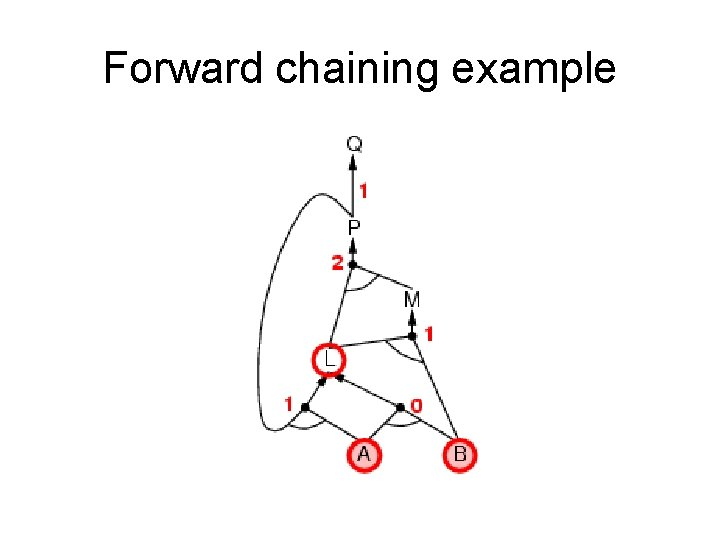Forward chaining example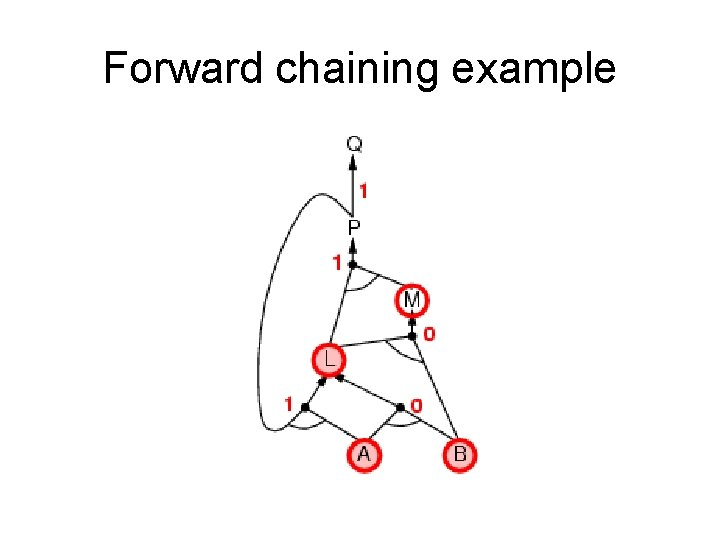Forward chaining example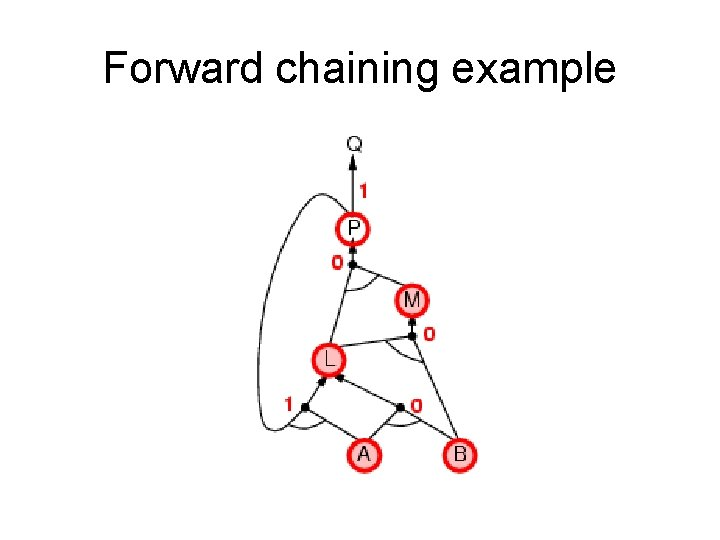Forward chaining example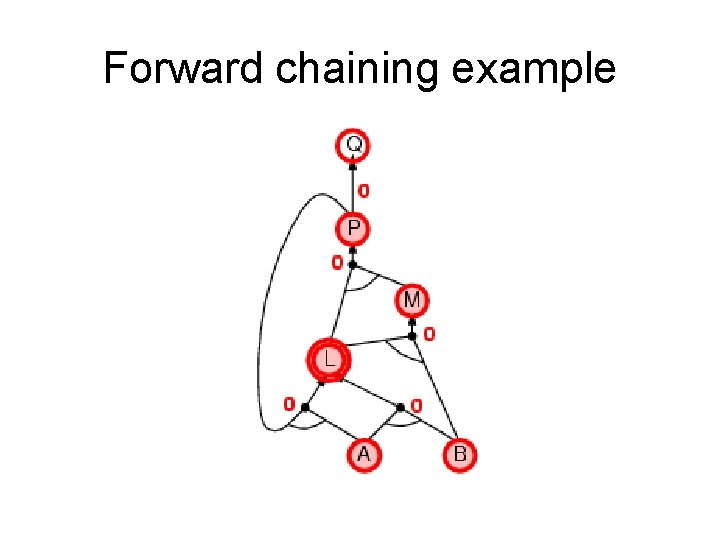Forward chaining exampleForward chaining exampleBackward chaining (BC) Idea: work backwards from the query q • • • check if q is known already, or prove by BC all premises of some rule concluding q Hence BC maintains a stack of sub-goals that need to be proved to get to q. Avoid loops: check if new sub-goal is already on the goal stack Avoid repeated work: check if new sub-goal 1. has already been proved true, or 2. has already failed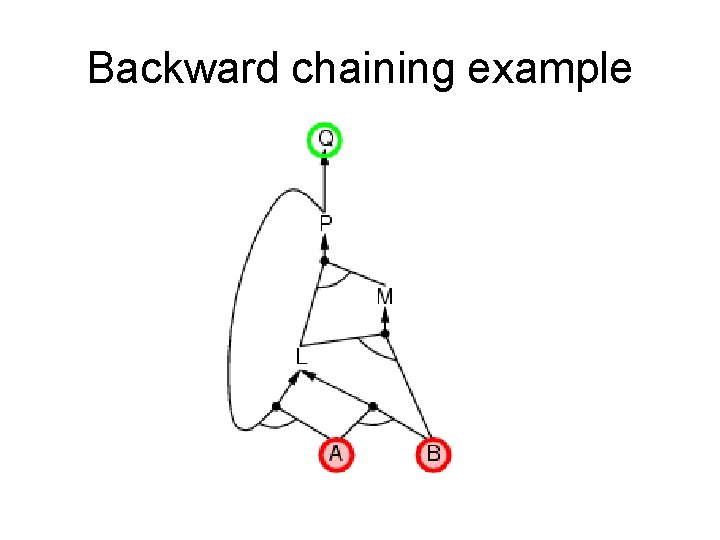Backward chaining exampleBackward chaining example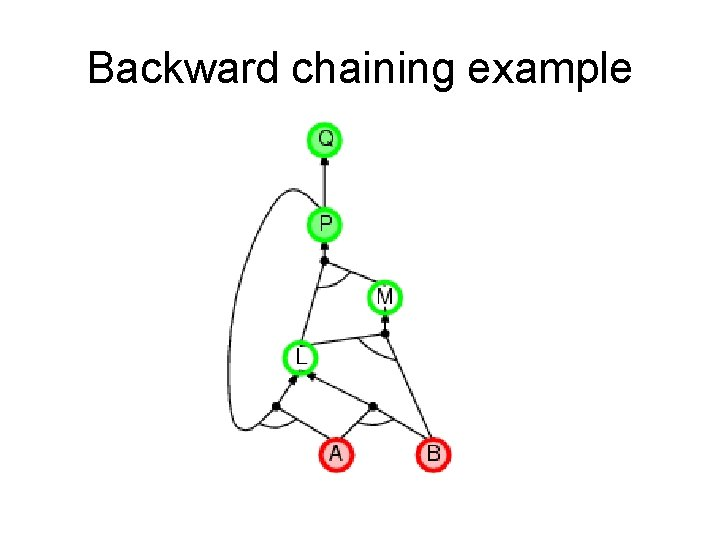Backward chaining example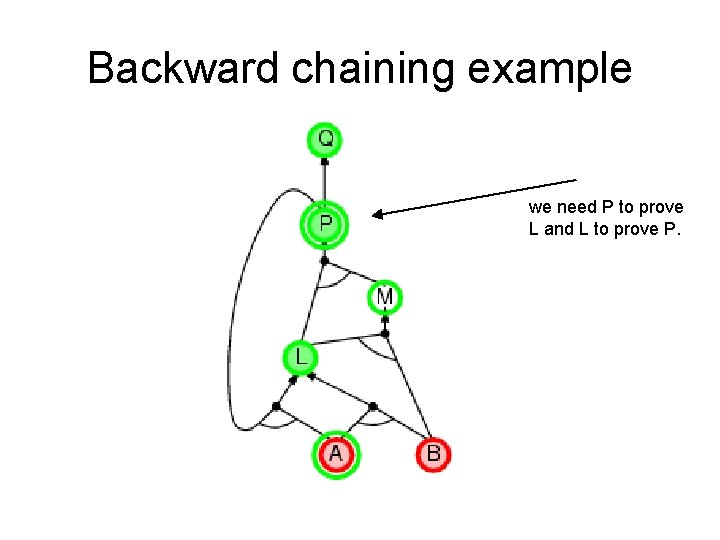Backward chaining example we need P to prove L and L to prove P.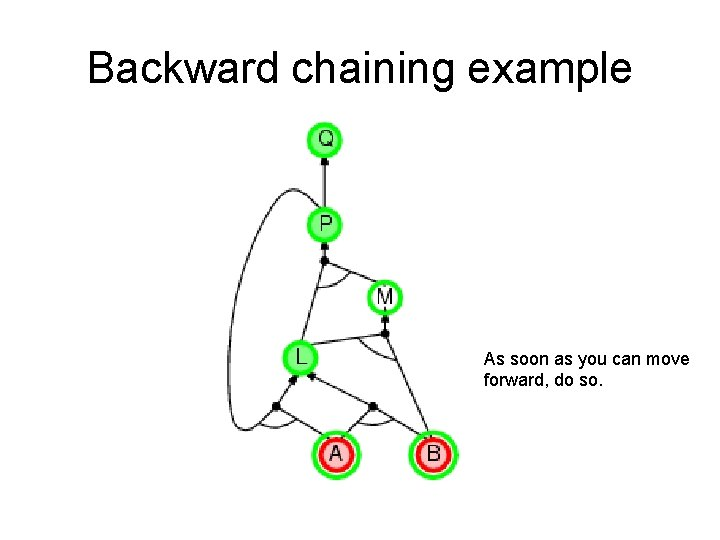Backward chaining example As soon as you can move forward, do so.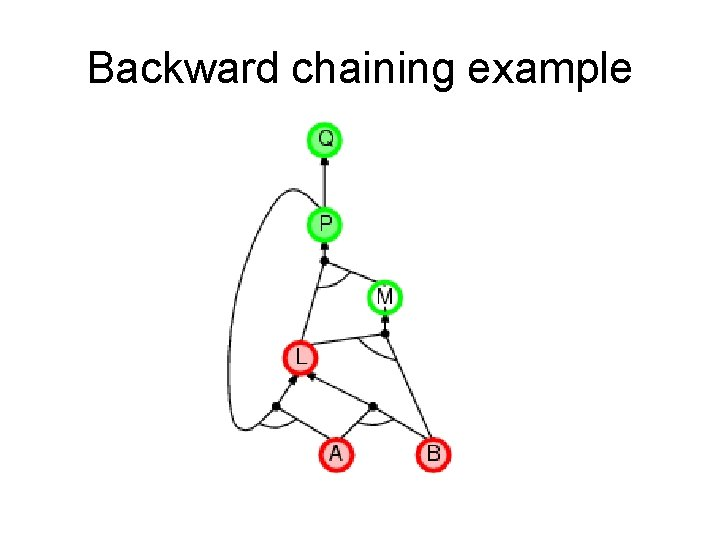Backward chaining example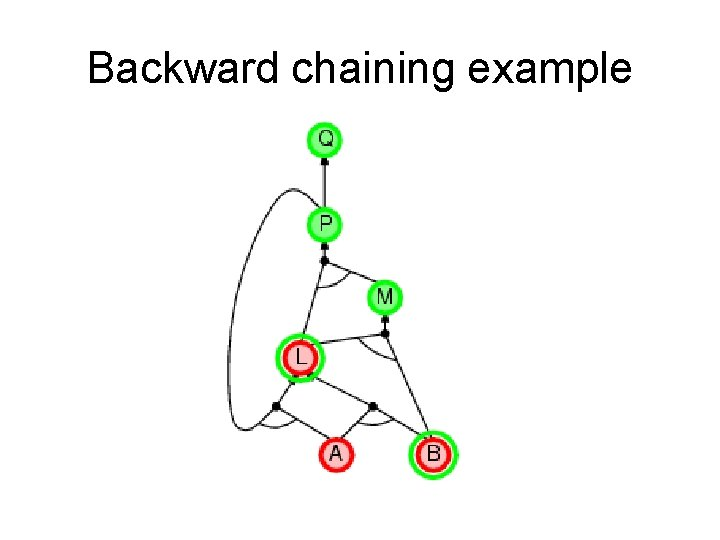Backward chaining example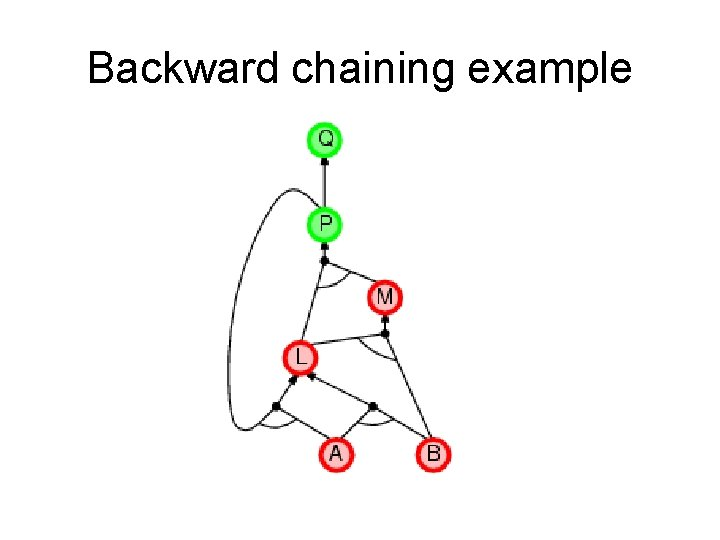Backward chaining example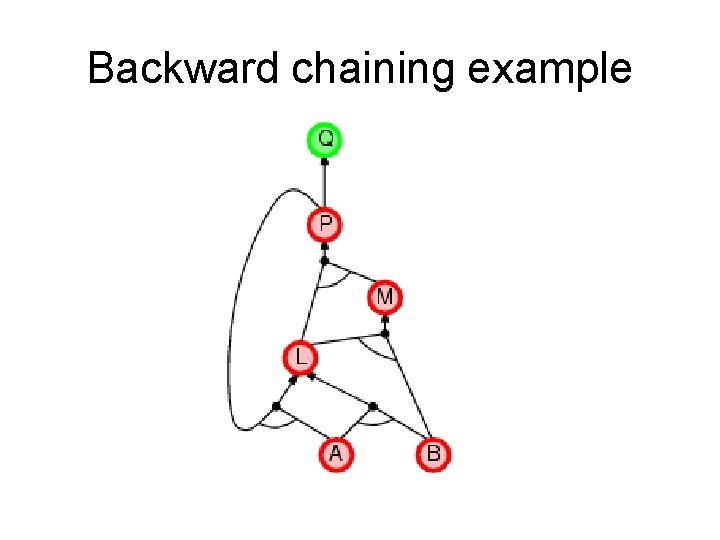Backward chaining example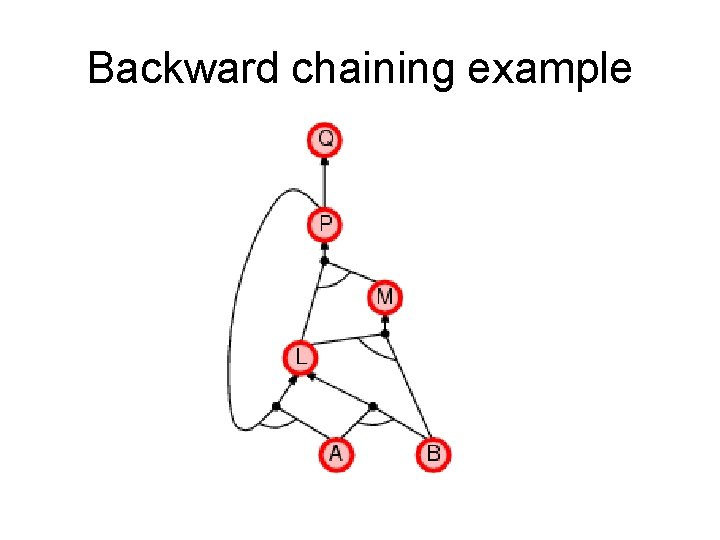Backward chaining exampleForward vs. backward chaining • FC is data-driven, automatic, unconscious processing, – e. g. , object recognition, routine decisions • May do lots of work that is irrelevant to the goal • BC is goal-driven, appropriate for problem-solving, – e. g. , Where are my keys? How do I get into a Ph. D program? • Complexity of BC can be much less than linear in size of KB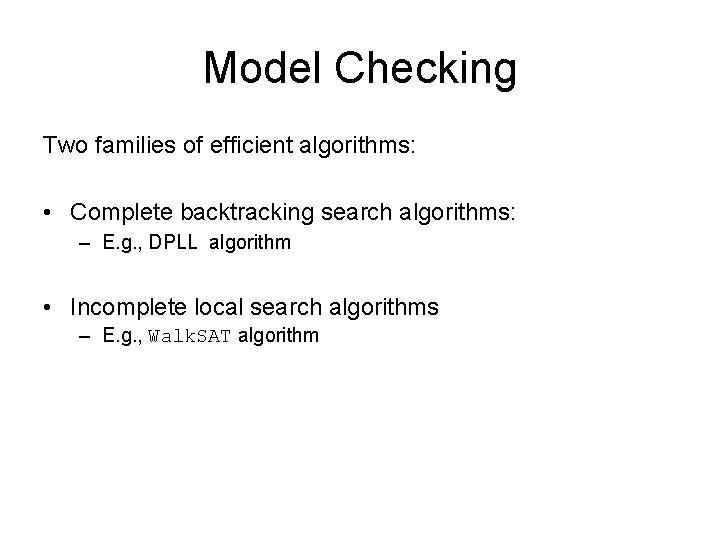Model Checking Two families of efficient algorithms: • Complete backtracking search algorithms: – E. g. , DPLL algorithm • Incomplete local search algorithms – E. g. , Walk. SAT algorithmThe DPLL algorithm Determine if an input propositional logic sentence (in CNF) is satisfiable. This is just backtracking search for a CSP. Improvements: 1. Early termination A clause is true if any literal is true. A sentence is false if any clause is false. 2. Pure symbol heuristic Pure symbol: always appears with the same "sign" in all clauses. e. g. , In the three clauses (A ∨ ¬B), (¬B ∨ ¬C), (C ∨ A), A and B are pure, C is impure. Make a pure symbol literal true. (if there is a model for S, then making a pure symbol true is also a model). 3 Unit clause heuristic Unit clause: only one literal in the clause The only literal in a unit clause must be true. Note: literals can become a pure symbol or a unit clause when other literals obtain truth values. e. g.The Walk. SAT algorithm • Incomplete, local search algorithm • Evaluation function: The min-conflict heuristic of minimizing the number of unsatisfied clauses • Balance between greediness and randomness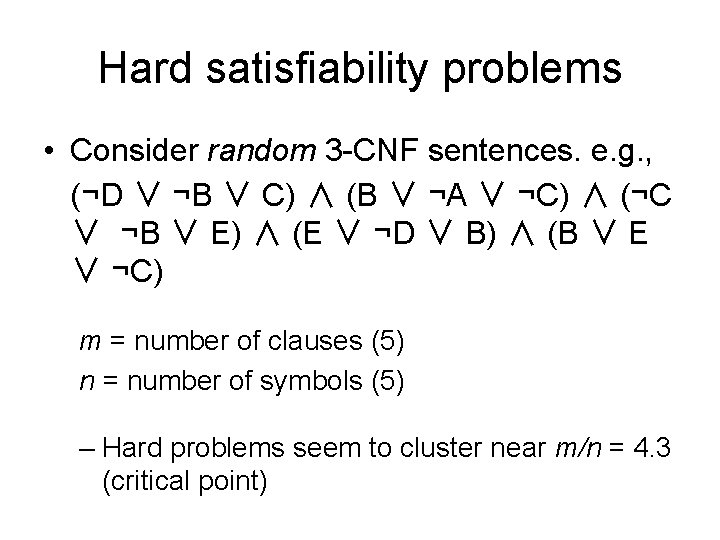Hard satisfiability problems • Consider random 3 -CNF sentences. e. g. , (¬D ∨ ¬B ∨ C) ∧ (B ∨ ¬A ∨ ¬C) ∧ (¬C ∨ ¬B ∨ E) ∧ (E ∨ ¬D ∨ B) ∧ (B ∨ E ∨ ¬C) m = number of clauses (5) n = number of symbols (5) – Hard problems seem to cluster near m/n = 4. 3 (critical point)Hard satisfiability problems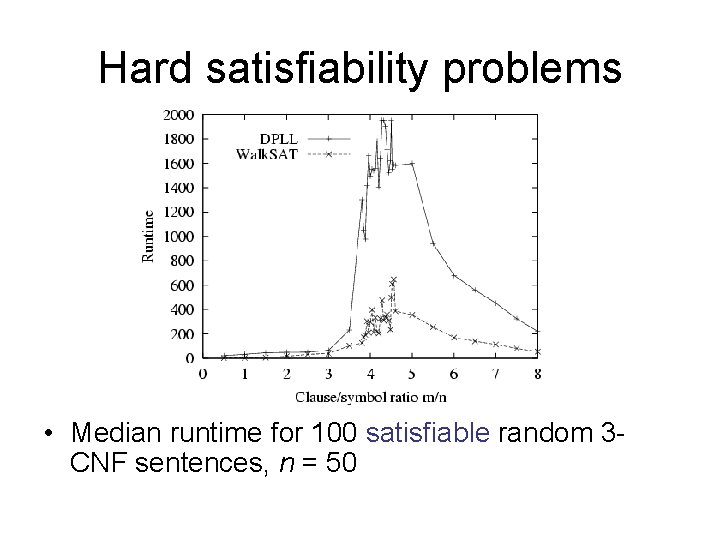Hard satisfiability problems • Median runtime for 100 satisfiable random 3 CNF sentences, n = 50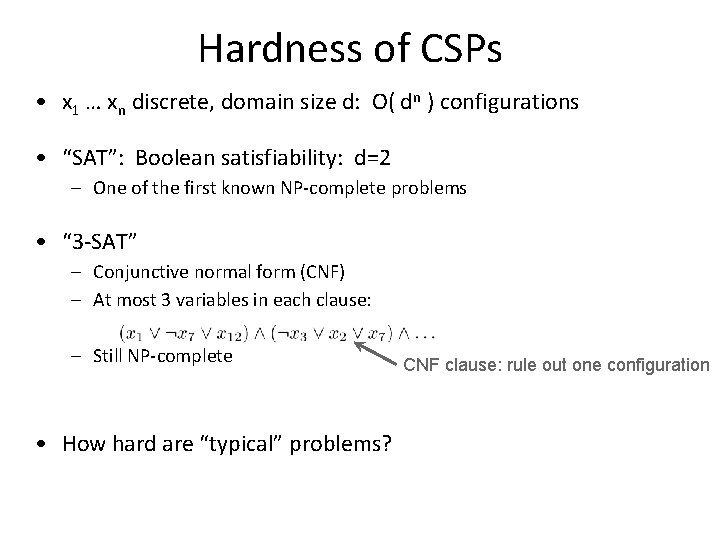Hardness of CSPs • x 1 … xn discrete, domain size d: O( dn ) configurations • “SAT”: Boolean satisfiability: d=2 – One of the first known NP-complete problems • “ 3 -SAT” – Conjunctive normal form (CNF) – At most 3 variables in each clause: – Still NP-complete • How hard are “typical” problems? CNF clause: rule out one configurationHardness of random CSPs • Random 3 -SAT problems: – n variables, p clauses in CNF: – Choose any 3 variables, signs uniformly at random – What’s the probability there is no solution to the CSP? – Phase transition at (p/n) ¼ 4. 25 – “Hard” instances fall in a very narrow regime around this point! avg time (sec) Pr[ unsat ] minisa t satisfiabl e ratio ( p/n ) unsatisifabl e easy, sat ratio ( p/n ) easy, unsat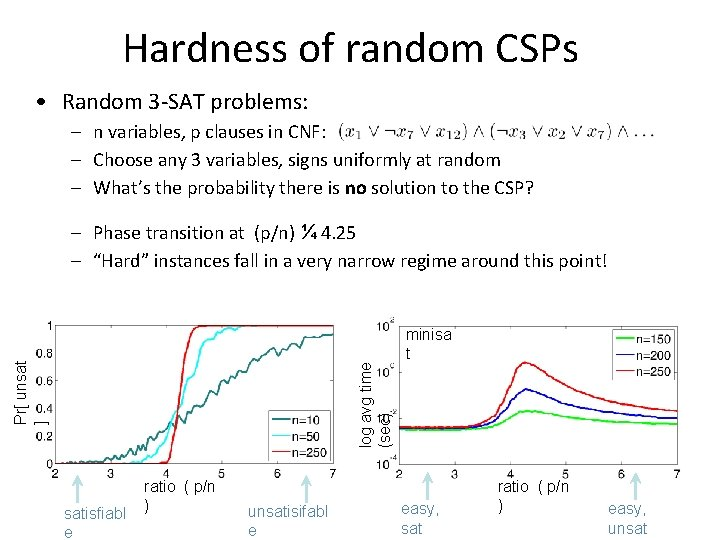Hardness of random CSPs • Random 3 -SAT problems: – n variables, p clauses in CNF: – Choose any 3 variables, signs uniformly at random – What’s the probability there is no solution to the CSP? log avg time (sec) Pr[ unsat ] – Phase transition at (p/n) ¼ 4. 25 – “Hard” instances fall in a very narrow regime around this point! satisfiabl e ratio ( p/n ) unsatisifabl e minisa t easy, sat ratio ( p/n ) easy, unsat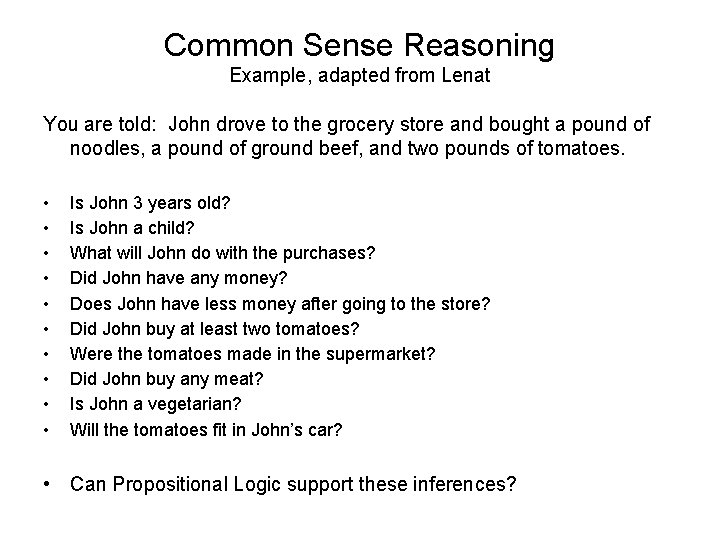Common Sense Reasoning Example, adapted from Lenat You are told: John drove to the grocery store and bought a pound of noodles, a pound of ground beef, and two pounds of tomatoes. • • • Is John 3 years old? Is John a child? What will John do with the purchases? Did John have any money? Does John have less money after going to the store? Did John buy at least two tomatoes? Were the tomatoes made in the supermarket? Did John buy any meat? Is John a vegetarian? Will the tomatoes fit in John’s car? • Can Propositional Logic support these inferences?Summary • Logical agents apply inference to a knowledge base to derive new information and make decisions • Basic concepts of logic: – – – syntax: formal structure of sentences semantics: truth of sentences wrt models entailment: necessary truth of one sentence given another inference: deriving sentences from other sentences soundness: derivations produce only entailed sentences completeness: derivations can produce all entailed sentences • Resolution is complete for propositional logic. Forward and backward chaining are linear-time, complete for Horn clauses • Propositional logic lacks expressive power